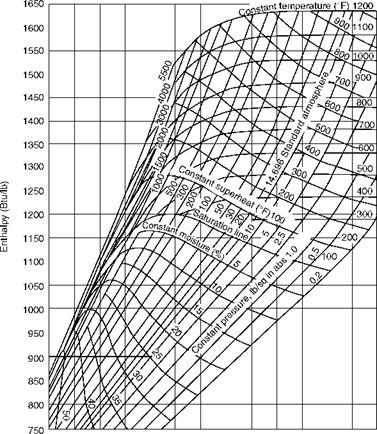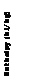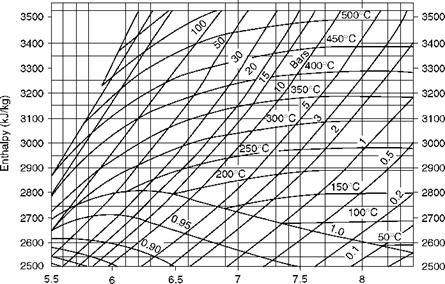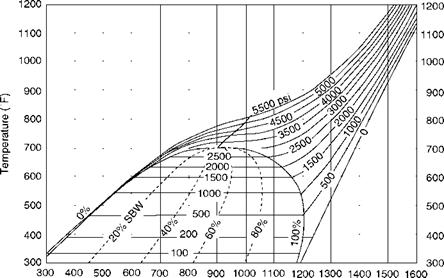## Temperature-Entropy (T-s) Diagram

The T-s diagram in imperial units is shown in Figure 2.7, which is used for plotting the steam cycle and understanding the way the steam by weight (SBW) increases as the water is heated. Refer to Chapter 9, where the water and steam conditions of a typical SC boiler are plotted on a T-s diagram in SI units.1.1 1.3 1.5 1.7 1.9 2.1 2.3 Entropy (Btu/lb °F) FIGURE 2.5 H-s or Mollier diagram in imperial units. (From Babcock and Wilcox Company, U. S.A. With permission.)

Entropy (kJ/kg K)

5.5 6 6.5 7 7.5 8

 Enthalpy (kJ/kg)Entropy (kJ/kg K)

 FIGURE 2.6 Mollier chart in SI units.

 Enthalpy (Btu/lb)

 FIGURE 2.7 T-s diagram for steam in British units. (From Babcock and Wilcox Company, U. S.A. With permission.)Комментирование и размещение ссылок запрещено.

Комментарии закрыты.

gazogenerator.com